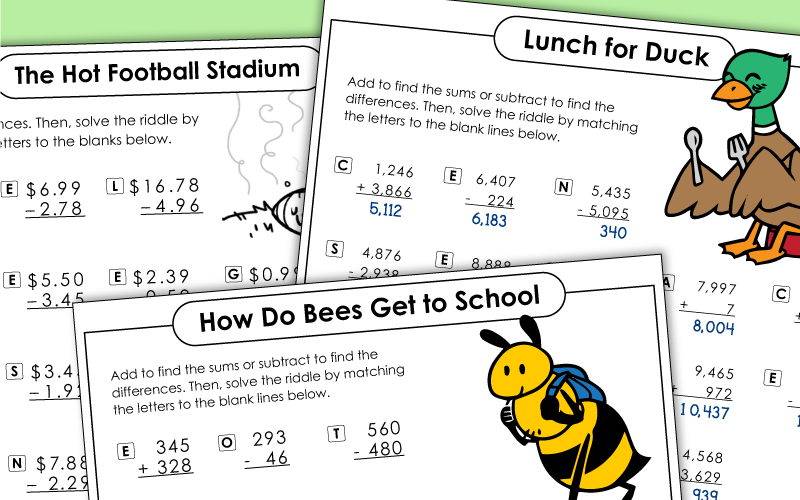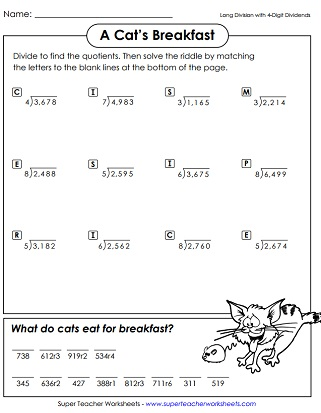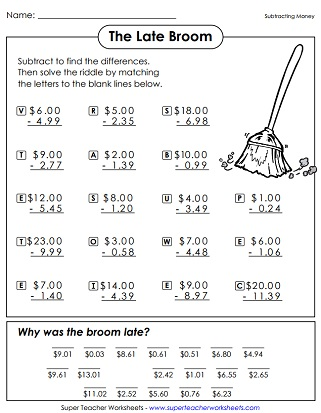# Math Riddle Worksheets

After solving a series of math problems, students are able to decode the answer to a funny riddle.What is a shark's favorite game? Solve the 3-digit addition (no regrouping) problems to find out.
What's more amazing that a talking dog? To find out, solve these 3-digit addition problems and decode the answer to the riddle. Each problem requires carrying or regrouping.
Why are there iron fences around graveyards? To find out, answer the addition mental math problems. (example: 3,000+2,000)
What do boats eat for breakfast? Find the sums by adding three addends together to see the answer.
Why did the cookie visit his doctor? The answer will make your students laugh out loud. Solve 4-digit addition problems to decode the punchline.
What do you call a skeleton who won't work? This worksheet has a set of tricky 4-digit column addition problems.
Did you hear the joke about the skunk? On this worksheet, students calculate the sums of two money amounts. Then they can use the sums to find the answer to the riddle.
Solve these fraction addition problems to find the answer to a fun riddle!

## Subtraction

Where did the farmer take his pony when it was ill? To find out, you'll have to solve two-digit subtraction problems. These problems do NOT require students to regroup/borrow.
What did Cinderella Fish wear to the Aquarium Ball? In order to solve the riddle, students will solve 3-digit subtraction problems. (None of these problems requires students to regroup.)
How do rabbits travel? To solve, complete a set of three-digit subtraction (with regrouping) math problems.
What did the alien say to the flowers in the garden? To find out, solve three-digit subtraction problems. Most problems require borrowing across zero.
Why was the football stadium so hot after the game? This riddle can be solved by subtracting pairs of money amounts.
Why was the broom late? To discover the answer to this riddle, kids must subtract pairs of money amounts.
Subtract hundreds and thousands on this mental math worksheet. Why was the music teacher locked out of her classroom? To find out, write the answer to each problem. (example: 9,000-4,000)

How do bees get to school in the morning? To find out, figure out the answers to the 3-digit addition and subtraction problems.
What did the duck eat for lunch? Solve 4-digit addition and subtraction problems. Then match the answers with the letters to see the answer to the riddle.
Solve the 5-digit addition and subtraction problems. Then match the letters to the answers to find the answer to this riddle: What are you supposed to do if you see an elephant that's blue?

## Multiplication

Do you know what a "twip" is? To find out, answer thirty-three basic multiplication facts.
Why did the girl eat her homework? To find out, write the answers to the basic multiplication facts.
Why did the cow do jumping jacks? Because she wanted to make a milkshake! To solve the riddle, students solve 2-digit by 1-digit multiplication problems.
Can you name the animal that jumps higher than a house? Complete the multiplication exercise to find out!
What did the doctor say when an invisible man walked into his office? To find the answer, multiply 3-digit and 1-digit numbers together.
Where do all the cows go on Friday nights? Multiply money amounts to complete the worksheet and find the answer. (example: \$7.63x7)
Why haven't you ever met anyone with a nose that's twelve inches long? Solve the multiplication facts to find out!
How do you know if your clock is crazy? To find out, solve these multiplication problems. Each one has a 2-digit factor and a 4-digit factor. (Example: 3,654 x 24)

## Division

What has four wheels and flies? This riddle worksheet can be solved by answering a series of basic division facts.
Why do sharks swim only in salt water? To see the solution to the riddle, find the missing dividend for each basic multiplication fact.
What makes a chess player really happy? Divide to find the 1-digit quotients with remainders. (example: 25/3 = 3r1)
What do you call a sleeping bull? To complete the math riddle, students solve division problems with three-digit dividends and 2-digit quotients. None of these problems have remainders.
What do you call a cow that's eating grass on your front lawn? Calculate the quotients. This one has 3-digit dividends with remainders.
What does a kitten eat for breakfast? The answer will have students laughing aloud. This math worksheet has long division with 4-digit dividends.

## Place Value

This printable has a series of numbers written out in word-name format. Where do dirty bats go to clean themselves? Students rewrite each number in standard form. Then match the numbers with the letters to find the answer to the laugh-out-loud riddle.
What did the teddy bear say when he finished his dinner? To solve the math riddle, kids will need to write the numbers in standard form.
Why was the six afraid of the seven? Write the value of the underlined digits to decode the riddle.
What do you call an anxious ogre? To find the funny answer to this riddle, simply write the numbers in standard form. Then match the letters and numbers to the blank lines at the bottom of the page.

## Rounding

Why did Humpty Dumpty have a great fall? Round each of the numbers to the nearest ten.
Why did the girl put cookies under her pillow? To find the answer, students must round 3 and 4-digit numbers to the nearest hundred.
Why did the bird get in trouble at school? For this one, kids will round money amounts to the nearest dollar.
Why did the elephant quit his job at the circus? To find out the answer to this silly riddle, students must round numbers to tens, hundreds, and thousands.

## Decimals

Convert mixed numbers to decimals. Then solve the riddle: Why did the clock get in trouble at school?
Convert mixed numbers to decimals. Then solve the riddle: Why did the banana have to see her doctor? Because she wasn't peeling very well.

## Integers

What has 13 hearts, but no other organs? To find out, add and subtract positive and negative integers.

## Order of Operations

This page contains a equations that can be solved with knowledge of the Order of Operations. This is a basic-level worksheet, with no parenthesis or exponents.
Students can solve 8 equations to demonstrate their understating of Order of Operations. This intermediate-level activity has problems that contain parenthesis, but no exponents.

## Basic Algebra

Why did the scarecrow win the Nobel Prize? Solve basic algebraic equations to find out. (example: 27 + x = 39)

## Roman Numerals

What did the ghost do when she hopped into her car? This printable math worksheet has roman numerals that students must convert to numbers in standard form.
Math Worksheets

We have thousands of math worksheets, covering a huge variety of topics. Includes operations, word problems, geometry, time, money, basic algebra, and much more!

Math Mystery Pictures

Solve the basic math problems (add, subtract, multiply, divide) to decode a color mystery picture.

## Worksheet ImagesMy Account
Site Information